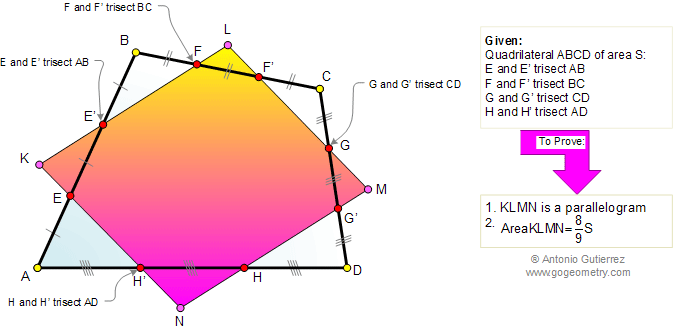Home Sitemap Geometry Problems All problems Parallel Area of a Triangle Segment Trisection Visual Index 551-560 Post a comment or solution
By Antonio Gutierrez
 Problem 558: Quadrilateral, Trisection, Sides, Wittenbauer Parallelogram, Area. Level: High School, College, Math Education The figure shows a quadrilateral ABCD. Points E, E', F, F', G, G', H, and H' trisect the sides AB, BC, CD, and AD, respectively. Extension of lines EH', E'F, F'G, and G'H meet at K, L, M and N, respectively. Prove that: (1) KLMN is a parallelogram known as Wittenbauer parallelogram; (2) Area of KLMN is 8/9 of area ABCD.Geometry problem solving Geometry problem solving is one of the most challenging skills for students to learn. When a problem requires auxiliary construction, the difficulty of the problem increases drastically, perhaps because deciding which construction to make is an ill-structured problem. By “construction,” we mean adding geometric figures (points, lines, planes) to a problem figure that wasn’t mentioned as "given." Recent Additions